Next: Langmuir Sheaths Up: Plasma Fluid Theory Previous: Drift Equations

# Closure in Collisionless Magnetized Plasmas

Up to now, we have only considered fluid closure in collisional magnetized plasmas. Unfortunately, most magnetized plasmas encountered in nature--in particular, fusion, space, and astrophysical plasmas--are collisionless. Let us consider what happens to the cold-plasma equations, the MHD equations, and the drift equations, in the limit in which the mean-free-path goes to infinity (i.e.,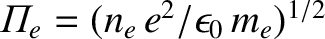).

In the limit, the cold-plasma equations reduce to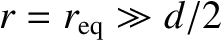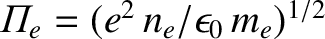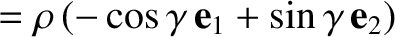(408)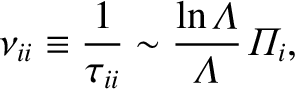(409)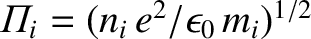(410)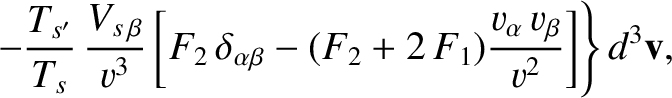Here, we have neglected the resistivity term, since it is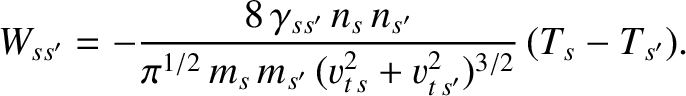. Note that none of the remaining terms in these equations depend explicitly on collisions. Nevertheless, the absence of collisions poses a serious problem. Whereas the magnetic field effectively confines charged particles in directions perpendicular to magnetic field-lines, by forcing them to execute tight Larmor orbits, we have now lost all confinement along field-lines. But, does this matter?

The typical frequency associated with fluid motion is the transit frequency,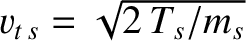. However, according to Eq. (345), the cold-plasma ordering implies that the transit frequency is of order a typical gyrofrequency: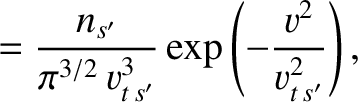(411)

So, how far is a charged particle likely to drift along a field-line in an inverse transit frequency? The answer is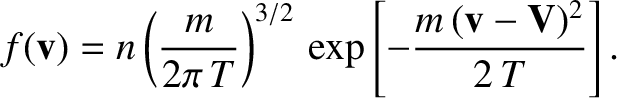(412)

In other words, the fluid motion in the cold-plasma limit is so fast that charged particles only have time to drift a Larmor radius along field-lines on a typical dynamical time-scale. Under these circumstances, it does not really matter that the particles are not localized along field-lines--the lack of parallel confinement manifests itself too slowly to affect the plasma dynamics. We conclude, therefore, that the cold-plasma equations remain valid in the collisionless limit, provided, of course, that the plasma dynamics are sufficiently rapid for the basic cold-plasma ordering (411) to apply. In fact, the only difference between the collisional and collisionless cold-plasma equations is the absence of the resistivity term in Ohm's law in the latter case.

Let us now consider the MHD limit. In this case, the typical transit frequency is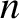(413)

Thus, charged particles typically drift a distance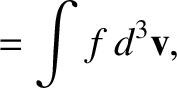(414)

along field-lines in an inverse transit frequency. In other words, the fluid motion in the MHD limit is sufficiently slow that changed particles have time to drift along field-lines all the way across the system on a typical dynamical time-scale. Thus, strictly speaking, the MHD equations are invalidated by the lack of particle confinement along magnetic field-lines.

In fact, in collisionless plasmas, MHD theory is replaced by a theory known as kinetic-MHD.The latter theory is a combination of a one-dimensional kinetic theory, describing particle motion along magnetic field-lines, and a two-dimensional fluid theory, describing perpendicular motion. As can well be imagined, the equations of kinetic-MHD are considerably more complicated that the conventional MHD equations. Is there any situation in which we can salvage the simpler MHD equations in a collisionless plasma? Fortunately, there is one case in which this is possible.

It turns out that in both varieties of MHD the motion of the plasma parallel to magnetic field-lines is associated with the dynamics of sound waves, whereas the motion perpendicular to field-lines is associated with the dynamics of a new type of wave called an Alfvén wave. As we shall see, later on, Alfvén waves involve the twanging'' motion of magnetic field-lines--a bit like the twanging of guitar strings. It is only the sound wave dynamics which are significantly modified when we move from a collisional to a collisionless plasma. It follows, therefore, that the MHD equations remain a reasonable approximation in a collisionless plasma in situations where the dynamics of sound waves, parallel to the magnetic field, are unimportant compared to the dynamics of Alfvén waves, perpendicular to the field. This situation arises whenever the parameter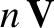(415)

(see Sect. 1.10) is much less than unity. In fact, it is easily demonstrated that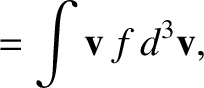(416)

where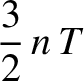is the sound speed (i.e., thermal velocity), andis the speed of an Alfvén wave. Thus, the inequality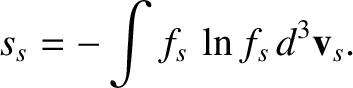(417)

ensures that the collisionless parallel plasma dynamics are too slow to affect the perpendicular dynamics.

We conclude, therefore, that in a low-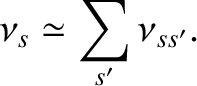, collisionless, magnetized plasma the MHD equations,(418)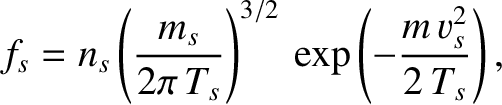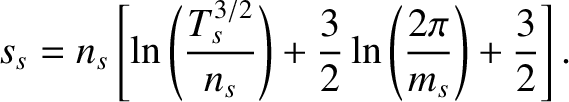(419)(420)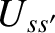(421)

fairly well describe plasma dynamics which satisfy the basic MHD ordering (413).

Let us, finally, consider the drift limit. In this case, the typical transit frequency is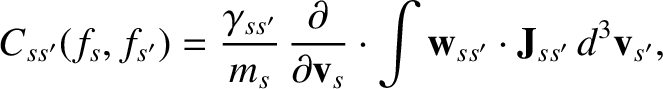(422)

Thus, charged particles typically drift a distance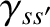(423)

along field-lines in an inverse transit frequency. In other words, the fluid motion in the drift limit is so slow that charged particles drifting along field-lines have time to traverse the system very many times on a typical dynamical time-scale. In fact, in this limit we have to draw a distinction between those particles which always drift along field-lines in the same direction, and those particles which are trapped between magnetic mirror points and, therefore, continually reverse their direction of motion along field-lines. The former are termed passing particles, whereas the latter are termed trapped particles.

Now, in the drift limit, the perpendicular drift velocity of charged particles, which is a combination of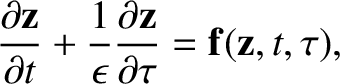drift, grad-drift, and curvature drift (see Sect. 2), is of order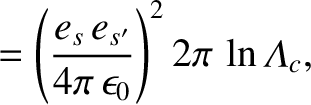(424)

Thus, charged particles typically drift a distance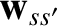(425)

across field-lines in an inverse transit time. In other words, the fluid motion in the drift limit is so slow that charged particles have time to drift perpendicular to field-lines all the way across the system on a typical dynamical time-scale. It is, thus, clear that in the drift limit the absence of collisions implies lack of confinement both parallel and perpendicular to the magnetic field. This means that the collisional drift equations, (391)-(394) and (394)-(397), are completely invalid in the long mean-free-path limit.

In fact, in collisionless plasmas, Braginskii-type transport theory--conventionally known as classical transport theory--is replaced by a new theory--known as neoclassical transport theory--which is a combination of a two-dimensional kinetic theory, describing particle motion on drift surfaces, and a one-dimensional fluid theory, describing motion perpendicular to the drift surfaces. Here, a drift surface is a closed surface formed by the locus of a charged particle's drift orbit (including drifts parallel and perpendicular to the magnetic field). Of course, the orbits only form closed surfaces if the plasma is confined, but there is little point in examining transport in an unconfined plasma. Unlike classical transport theory, which is strictly local in nature, neoclassical transport theory is nonlocal, in the sense that the transport coefficients depend on the average values of plasma properties taken over drift surfaces. Needless to say, neoclassical transport theory is horribly complicated!Next: Langmuir Sheaths Up: Plasma Fluid Theory Previous: Drift Equations
Richard Fitzpatrick 2011-03-31